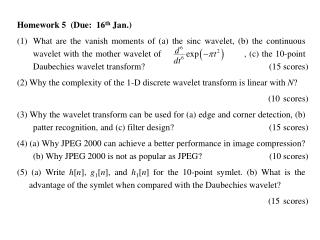DownloadDownload PresentationHomework 5 (Due: 16 th Jan.)

# Homework 5 (Due: 16 th Jan.)

Download Presentation## Homework 5 (Due: 16 th Jan.)

- - - - - - - - - - - - - - - - - - - - - - - - - - - E N D - - - - - - - - - - - - - - - - - - - - - - - - - - -
##### Presentation Transcript

1. Homework 5 (Due: 16thJan.) What are the vanish moments of (a) the sinc wavelet, (b) the continuous wavelet with the mother wavelet of , (c) the 10-point Daubechies wavelet transform? (15 scores) (2) Why the complexity of the 1-D discrete wavelet transform is linear with N? (10 scores) (3) Why the wavelet transform can be used for (a) edge and corner detection, (b) patter recognition, and (c) filter design? (15 scores) (4) (a) Why JPEG 2000 can achieve a better performance in image compression? (b) Why JPEG 2000 is not as popular as JPEG? (10 scores) (5) (a) Write h[n], g1[n], and h1[n] for the 10-point symlet. (b) What is the advantage of the symlet when compared with the Daubechies wavelet? (15 scores)

2. (6) (a) Write a Matlab program for the following 2-D discrete 8-point Daubechies wavelet. [x1L, x1H1, x1H2, x1H3] = wavedbc8(x) g[m]  2 x1,L[m, n] along m g[n]  2 v1,L[m, n] along n h[m]  2 x1,H1[m, n] x[m, n] along m x1,H2[m, n] g[m]  2 h[n]  2 v1,H[m, n] along m along n  2 x1,H3[m, n] h[m] along m (b) Also write the program for the inverse 2-D discrete 8-point Daubechies wavelet transform. x = iwavedbc8(x1L, x1H1, x1H2, x1H3) The Matlab program should be mailed to me. (35 scores)